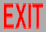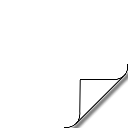# Twistor superstrings

(talk at Simons Workshop in Mathematics and Physics 2004)

## ADHM supertwistors

### Projective lightcone

Manifest symmetry (representation, not nonlinear realization) is important for simplification, to make things both clearer and easier to calculate.

Before Penrose, there was Dirac: for coordinate representation of D-dimensional conformal group SO(D,2) by (D+2)-vector y instead of spinor, impose 3 equations on fields:

• y2 = 0             constrains to cone to kill 1 coordinate
• y∙∂/∂y = 0     projective to kill another
• (∂/∂y)2 = 0     gives usual Klein-Gordon (massless)
In lightcone notation (dy2 = -2dy+dy- + rest),

y = y+(1,½x2,x)     →     dy2 = (y+)2dx2:     conformally flat

(For a particle, (y+)2 becomes the worldline metric.)
Projective lightcone is (degenerate) zero-radius limit of (A)dS
(e.g., in AdS/CFT, AdS5 → flat 4D space: instant holography)

### Penrose vs. ADHM

To avoid problems of reality, etc., spacetime signature = (--++)
→ superconformal group: SL(4|N) for N supersymmetries
(For strings, N=4 → PSL(4|4) = SL(4|4)/GL(1))

2 kinds of (super)twistors:

• Penrose (original)
• Atiyah-Drinfel'd-Hitchin-Manin (instanton)

Penrose ADHM RP(3|N) → 3 bosons + N fermions on shell HP(1|½N) → 4 bosons + 2N fermions off shell defining: ΛA /GL(1) A = (4|N) of SL(4|N) SL(4|N): GAB = ΛBΛA - tr GL(1): H = ΛAΛA "flag": Λα'A /GL(2) α' = 2 of GL(2) SL(4|N): GAB = Λα'BΛα'A - tr GL(2): Hα'β' = Λβ'AΛα'A Lorentz SO(2,2) = SL(2)2 4-momentum pαα̇ put on mass(less) shell: p2 = det(pαα̇) = 0 → pαα̇ = λαλα̇ → (λα, λα̇)/GL(1) conformal SO(3,3) = SL(4) 6-vector y[ab] put on projective lightcone: y2 = Pf(y[ab]) = 0 (& y∙∂/∂y = 0) → y[ab] = λα'aλα'b → λα'a/GL(2) (super: y[AByCD) = 0, etc.)
(Can generalize GL(2) → GL(2|n), but not useful for selfduality)

Metric in terms of ADHM twistors (D=4):

dy2 = dλα'aβ'bα'cλβ'dεabcd)

### Signature

Other than (--++) is more complicated:

conformal covering reality Penrose SO(6) SO(5,1) SO(4,2) SO(3,3) SU(4) SU*(4) SU(2,2) SL(4) C P(seudo) C R ✓ X ✓ ✓ X P: SU(2) X R: SL(2) ++++ -+++ --++

Penrose needs real phase space, with momenta dual to coordinates
AΛA = R⊕R or C⊕C*);
ADHM needs real coordinate space
α'A = R⊗R or P⊗P).

So both twistors work in (--++), Penrose also in (-+++) (as invented), ADHM also in (++++) (as invented for instantons).
Complex for either ("X") → doubling (or no reality).

### Where in twistorspace is x?

Penrose twistors defined in momentum space; get to coordinate space by Fourier → Penrose transform:

φ(x) = ∫dp φ̃(p) eix∙p = ∫dλ dλ φ̂(λ,λ) eiλxλ = ∫dλ φ̌(λ,λx) = ∫dλ dμ δ2(μ-λx) φ̌(λ,μ)

where ΛA = (λαα̇), ΛA = (μ̄α,λα̇) (and similar for N>0). Thus

Λα̇ = Λαxαα̇

On the other hand, ADHM twistors are already in coordinate space (momentum space is not so useful for nonperturbative solutions):

Λβ'A = Λβ'γ ( δγα, xγα̇, θγa)
A = (α,α̇,a) as SL(4|N) ⊃ SL(2)2SL(N) (superconformal ⊃ Lorentz ⊗ internal)

So we have in particular

Λβ'α̇ = Λβ'αxαα̇

Similar to Penrose, but invertible for x;
to get coordinates of chiral superspace (x,θ), do one of:

• GL(2) gauge Λβ'γ = δβ'γ
• use constrained projective lightcone coordinates yAB = Λγ'AΛγ'B
• use HP(1|½N) projective coordinates (xβα̇βa) = Λ-1βγ'γ'α̇γ'a)
Chiral superspace has no torsion.

### Field equations

Free field equations in terms of superconformal generators;
generalization of p2 = 0 (Gα̇β = pβα̇):

G[A[CGB)D) - tr = 0                 [   ) = graded antisymmetrize

Satisfied trivially by Penrose; for ADHM, →

Λα'AΛα'B = 0

Field equations for free scalar (Λ → -i∂/∂Λ):

α'Aα'B φ = 0

After GL(2) gauge fixing, just truncate

A → (α̇,a):     ∂ → (∂/∂x,∂/∂θ)

∂∂ = 0:

• (∂/∂x)2 = 0         (Klein-Gordon)
• (∂/∂x)(∂/∂θ) = 0     (κ symmetry)
• (∂/∂θ)2 = 0
Free "lightcone" solution takes ADHM → Penrose (cf. p2 = 0):

Λα'A = Λα'ΛA
"gauge" Λα' = δα'+

This allows manifestly superconformal generalization of Penrose transform:
GL(2) says momentum-space wave functions are independent of Λα'

 φ(Λα'A) = ∫dΛA dΛα' exp(iΛα'AΛα'ΛA) φ̂(ΛA) = ⎧⎨⎩ ∫dΛA δ2(Λα'AΛA) φ̂(ΛA) ∫dΛα' φ̌(Λα'AΛα')
which reduces to the usual in the gauge Λβ'γ = δβ'γ

Selfdual Yang-Mills in terms of ∇α'A = ∂α'A + iAα'A:

[∇α'A,∇β'B} = Cα'β'FAB

where Cα'β' = - Cβ'α' is the SL(2) metric.
Truncation A → (α̇,a) gives usual chiral superspace equations.

The ADHM solution writes Aα'A in terms of matrices that are proportional to Λ.

## ADHM twistor superstrings

### Topological

Berkovits formulation of Nair/Witten twistor superstring, as closed string:

L = (∇-ΛA)Λ-A + LYM

- = right-handed worldsheet derivative, covariant with respect to 2D:

• coordinate
• Lorentz
• twistor GL(1)

LYM is for Yang-Mills symmetry current.
3 important ingredients:

• supertwistors: take place of x
• Yang-Mills current: Yang-Mills group theory, denominators of amplitudes
• GL(1) worldsheet "instantons": perturbative helicity expansion about selfdual Y-M

This action follows as lightcone solution (see above) of ADHM twistor superstring:

L' = (∇-Λα'A)Λ-α'A + g-α'β'Λβ'AΛ-α'A + g--ABΛ-α'AΛ-α'B + LYM

where now ∇ is not GL(1), which is part of GL(2) gauge field g-α'β'.
Two kinds of constraints imposed by gauge fields:

• g-α'β':     GL(2), kills extra coordinates
• g--AB:     field equations (K-G, etc.)
Simultaneously has usual x and GL(1) for instantons

### Feynman diagrams

Second-quantization gives Feynman diagrams, graphs associated with mathematical expressions for scattering amplitudes, given in coordinate space by associating a "vertex factor" with each vertex (an interaction point in space), and a propagator (Green function for the wave equation) with each line.

To relate to first-quantization, we'll consider the fields to be scalars, carrying no Lorentz indices (which may be hidden if the coordinates include fermions). However, they will carry internal symmetry indices, by making the scalars N×N matrices. (From now on, N ≠ # of supersymmetries.) In 't Hooft's notation (inspired by string theory), this is indicated by replacing lines with double lines, which are continuous (no branches, and ending only on asymptotic states), reflecting the N-fold symmetry of the action, which has an overall trace.

This notation gives a 2-dimensionality to Feynman graphs, by filling in the space between lines, and also that inside closed loops of such lines. Such closed loops get factors of N from summing over the internal symmetry index, and by Euler's theorem any graph then gets a factor of

(g2)ℓ-1NF = (Ng2)ℓ-1N-2(h-1)     [P-V-F = 2(h-1),     P-V = ℓ-1   →   F = ℓ-1 -2(h-1)]

for ℓ loops of the original lines, F faces (P propagators & V vertices), and h handles (genus), where g is the coupling, which appears in the action as an overall 1/g2 (= 1/ħ).

### Random lattice

The above 2nd-quantized Feynman diagram surface (polyhedron) can be associated with a 1st-quantized string amplitude (Nielsen, Olesen, Fairlie, Sakita, Virasoro, Douglas, Shenker, Gross, Migdal, Brézin, Kazakov), the path integral of e-S, as:
• polyhedron = "random" lattice (discretization) of worldsheet
• sum over Feynman diagrams = integral over worldsheet metrics
• 1/N that counts genus = string coupling
• Ng2 that counts loops = - exp( - coefficient of worldsheet cosmological term )
• Feynman propagator = exp( - worldsheet action for x )
For the usual string, the x part of the action is latticized as

½∫(∂x)2/α' → ½∑<ij> (xi - xj)2/α'

for string "slope" α' and links <ij> (lines), which gives the unusual propagator

Δ = exp(-x2/2α')

which accounts for several of the unusual features of known string theories (those that don't seem to apply to hadrons).

### Wrong-sign φ4

For physical "partons", we want (for massless)

Δ = 1/x2

in D=4, or other powers in D≠4 from Fourier transforming 1/p2. However, note that "T-duality" (symmetry under Fourier transformation) requires D=4 for this propagator (but no restriction for the Gaussian one).

On the other hand, we need an exponential propagator for e-S, so use

1/x2 = ∫0 dτ exp( -τx2 )

The discretized x action is then

½∑<ij> τij(xi - xj)2

Note, e.g., this means 2 components for τ at each vertex on a regular (flat) square lattice. We can enforce this in general by making τ a traceless tensor: In the continuum limit,

S → ½∫τ±±(∇±x)2

Sometimes we prefer a 1st-order formalism, where

L = (∇±x)∙p± -½(τ±±)-1(p±)2

Since τ is associated with lightlike directions, the lattice is thus a lightlike lattice, with any vertex having 4 lightlike directions, corresponding to φ4 theory.

In field theory, a coupling G in the action gives a factor -G at a vertex, because of exp(-S2) for 2nd-quantized S2 when perturbatively expanding in the interaction part of -S2. But the strings 1st-quantized action S1 always gives positive amplitudes because exp(-S1) is always positive. Thus, G is always negative in this correspondence. In this case, we then have "wrong-sign" Gφ4=g2φ4 theory (potential unbounded below). However, in D=4 this theory (because of the wrong sign) is "asymptotically free": better behaved perturbatively.

### QCD superstring?

Twistor superstrings aren't stringy (no excited states). One way to reduce strings to particles is limits on tension (inverse of slope α'):
• α' → 0:   truncation to massless states
• α' → ∞:   no tension; string "falls apart" into constituent partons
They're taken after rescaling 1 worldsheet coordinate by α', so α' → 0 collapses the string in that direction, while α' → ∞ expands it to ∞. The latter limit can be taken explicitly on a sting action. It leads to a degenerate worldsheet metric: The worldsheet breaks up into worldlines. There are 2 possible degenerate directions:
• spacelike:   (∂x)2 → ẋ2   (usual)
• lightlike:   (∂x)2 → 0,   but   τ±±(∇±x)2 → τ--(∇-x)2   (chiral)
(Not timelike, since then the partons would not propagate to σ0 = ±∞.) Thus, the chiral action of the twistor superstring can come as a tensionless limit only from a string with ordinary parton propagators: Since both relate to QCD, this is not totally unexpected. Working backwards, we then find the stringy twistor superstring action

L = (∇±Λα'A)Λ±α'A + g±α'β'Λβ'AΛ±α'A + g±±ABΛ±α'AΛ±α'B + LYM

where LYM now also has ± terms.